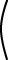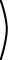```0.5 0.0 0.0 0.0 0.5 0.0 0.0 0.0 0.5 ``````2 4 6 0 -12 -8 4 0 4 ```= (0.5)I```2 4 6 0 -12 -8 4 0 4 ```=

 0.5```2 4 6 0 -12 -8 4 0 4 ```=```1 2 3 0 -6 -4 2 0 2 ```# Diagonal Matrix

The diagonal matrix (aI) is sometimes useful in computer graphics. Say for example that what you want to compute is:

a Ax

where a is a scalar and x is a column matrix. Sometimes it is convenient to think of this as:

(aI)Ax

Now every operation is a matrix multiplication. This is an advantage since matrix multiplication can be done by graphics hardware.

### QUESTION 7:

What is (2I)(0.5I)?